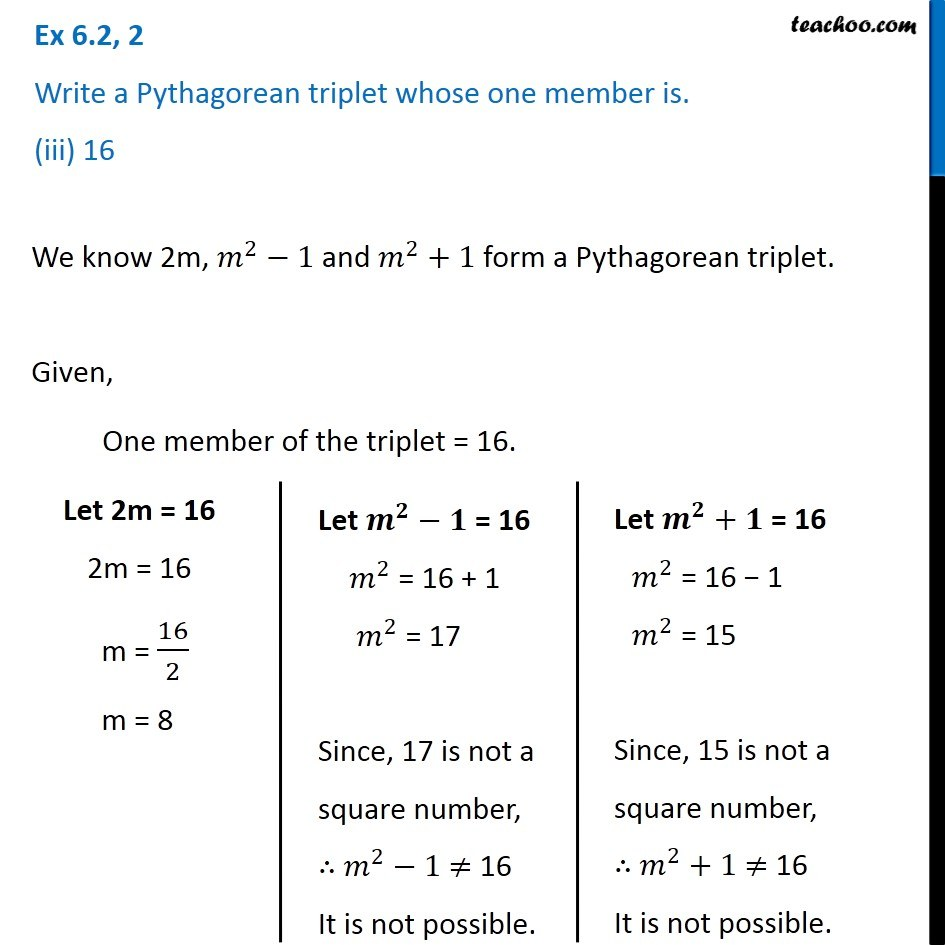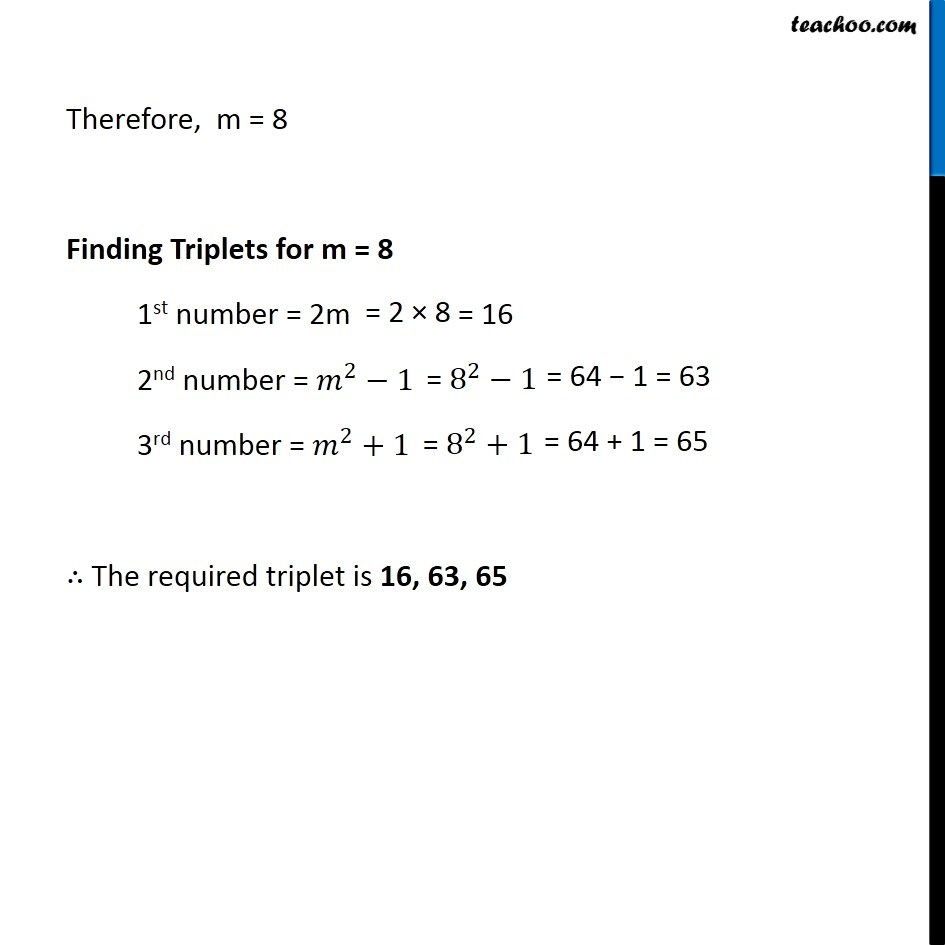Subscribe to our Youtube Channel - https://you.tube/teachoo

1. Chapter 6 Class 8 Squares and Square Roots
2. Concept wise
3. Pythagorean triplets

Transcript

Ex 6.2, 2 Write a Pythagorean triplet whose one member is. (iii) 16We know 2m, 𝑚^2−1 and 𝑚^2+1 form a Pythagorean triplet. Given, One member of the triplet = 16. Let 2m = 16 2m = 16 m = 16/2 m = 8 Let 𝒎^𝟐−𝟏" = 16" 𝑚^2 = 16 + 1 𝑚^2 = 17 Since, 17 is not a square number, ∴ 𝑚^2−1 ≠ 16 It is not possible. Let 𝒎^𝟐−𝟏" = 16" 𝑚^2 = 16 + 1 𝑚^2 = 17 Since, 17 is not a square number, ∴ 𝑚^2−1 ≠ 16 It is not possible. Therefore, m = 8 Finding Triplets for m = 8 1st number = 2m 2nd number = 𝑚^2−1 3rd number = 𝑚^2+1 ∴ The required triplet is 16, 63, 65

Pythagorean triplets

About the AuthorDavneet Singh
Davneet Singh is a graduate from Indian Institute of Technology, Kanpur. He has been teaching from the past 9 years. He provides courses for Maths and Science at Teachoo.